DraftKings NFC Championship Odds
+250
2.5 to 1Buccaneers
25.4% implied probability

+400
4 to 1Rams
17.8% implied probability

+450
4.5 to 1Cardinals
16.1% implied probability

+600
6 to 1Cowboys
12.7% implied probability

+600
6 to 1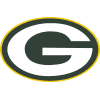Packers
12.7% implied probability

+1800
18 to 1Saints
4.7% implied probability

+3500
35 to 1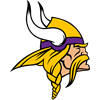Vikings
2.5% implied probability

+4000
40 to 149ers
2.2% implied probability

+5000
50 to 1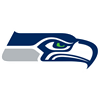Seahawks
1.7% implied probability

+8000
80 to 1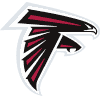Falcons
1.1% implied probability

+10000
100 to 1Panthers
0.9% implied probability

+10000
100 to 1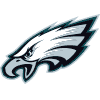Eagles
0.9% implied probability

+20000
200 to 1Bears
0.4% implied probability

+20000
200 to 1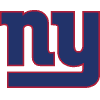Giants
0.4% implied probability

+20000
200 to 1Football Team
0.4% implied probability

+50000
500 to 1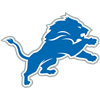Lions
0.2% implied probability